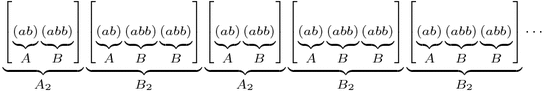# The “Fibonacci”est String

The celebrated Fibonacci Sequence is defined by setting each term equal to the sum of the two previous terms, starting at $$F_0=0$$ and $$F_1=1$$. So it continues like this:

0, 1, 1, 2, 3, 5, 8, 13, 21, 34, 55, 89, 144, 233, 377, …

What’s the relation to last week‘s infinite jump sequence,

a b a b b a b a b b a b b a b a b b a b a b b a b b a b a b b a b b … ?

Let’s find out!

Recall from last week that we can group our sequence into blocks of $$A=(ab)$$ and $$B=(abb)$$, and that the pattern of “short” and “long” blocks is the same as the pattern of as and bs in the original sequence:This means we can do this grouping again! Specifically, we can group our sequence into “blocks of blocks”, or level 2 blocks, that look like $$A_2=[(ab)(abb)]$$ and $$B_2=[(ab)(abb)(abb)]$$:Furthermore, the pattern of short and long level 2 blocks is still the same as the original jump sequence! So we can keep going, grouping our sequence into level 3 blocks $$A_3=A_2 B_2$$ and $$B_3=A_2 B_2 B_2$$, level 4 blocks $$A_4=A_3 B_3$$ and $$B_4=A_3 B_3 B_3$$, and so on, thus finding longer and longer patterns that fill up our sequence. Here’s level 3:One natural question is: what are the lengths of these blocks? Let’s make a table:

n An Bn An Length Bn Length
0 a b 1 1
1 ab abb 2 3
2 ababb ababbabb 5 8
3 ababbababbabb ababbababbabbababbabb 13 21

The lengths are exactly the Fibonacci numbers! (Prove this!) Furthermore, how many as and bs does each block have? Here’s another table:

n An # of as # of bs Bn # of as # of bs
0 a 1 0 b 0 1
1 ab 1 1 abb 1 2
2 ababb 2 3 ababbabb 3 5
3 ababbababbabb 5 8 ababbababbabbababbabb 8 13

Again the Fibonacci numbers! This gives us another way to compute the ratio of as to bs in the jump sequence: The ratios of bs to as in blocks An and Bn are $$F_{2n}/F_{2n-1}$$ and $$F_{2n+1}/F_{2n}$$ respectively, so as n gets larger these ratios should both approach last week’s answer, the golden ratio $$\phi$$.

OK, so our jump sequence is very “Fibonacci”y: it has blocks of Fibonacci lengths with Fibonacci proportions of as and bs. How can it get any “Fibonacci”er than that? Here’s how: Define a different infinite string using the same definition as the Fibonacci numbers themselves: write $$W_0=0$$, $$W_1=1$$, and after that, each string is formed by writing the previous two next to each other: $$W_n=W_{n-1}W_{n-2}$$. The Ws continue like this:

0, 1, 10, 101, 10110, 10110101, 1011010110110, 101101011011010110101, …,

and in the limit they define some infinite string of 0s and 1s that, without a doubt, is as “Fibonacci”y as it gets. But wait; except for the first digit, this is exactly the same as our jump sequence with 0=a and 1=b!
- 1 0 1 1 0 1 0 1 1 0 1 1 0 1 0 1 1 0 1 0 1 1 0 1 1 0 1 0 1 1 0 1 1 ... a b a b b a b a b b a b b a b a b b a b a b b a b b a b a b b a b b ... This truly is the Fibonacci numbers reincarnated in a binary string.

## 4 thoughts on “The “Fibonacci”est String”

1.Michael Paul Goldenberg says:

Another vote for Joe Mazur’s books, who is the brother of renowned mathematician, Barry Mazur, by the way. Also a neighbor in Vermont of a couple of very close friends of mine, one of whom is the daughter of the late French number theorist and founding member of Bourbaki, Andre Weil.

2.Michael P says:

Hey Zach,

I’d love to know where you get your ideas for your posts. Are there any books that you’d recommend? Are these things you discover yourself through your own research?

Thanks!

1.Zachary Abel says:

Michael,

Good question! I try to blog about facts or topics that (a) I delighted in learning, seeing, or discovering; (b) I think my target audience will “get” and find surprising, beautiful, or mind-bending; (c) and can be explained in “bite-sized chunks” with as little assumed knowledge as possible.

For part (a), I don’t restrict myself to any particular source. For example, Voronoi regions are a classic topic in my research field, while the grid problem you commented on (thanks, by the way!) I originally saw while training for high school math competitions.

For parts (b) and (c) I often take cues from undergraduate dining hall conversations, through questions someone asks or cool factoids that they or I bring to the table (hah!). Consider this “market research”. =D

As for books, I think Euclid in the Rainforest: Discovering Universal Truth in Logic and Math by Joseph Mazur is relevant and right up your alley. It ties classical mathematical problems and themes into a fun narrative exploring math from an epistemological and didactic perspective.

1.Michael P says: